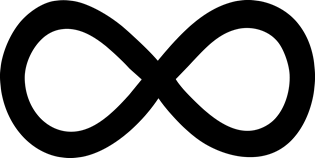# Infinity

In Mathematics, “infinity” is the concept describing something which is larger than the natural number. It generally refers to something without any limit. This concept is predominantly used in the field of Physics and Maths which is relevant in the number of fields. For the extended real number system, the term “infinity” can be also be used.

## What is Infinity?

Infinity is an idea of something that has no end. In general, it is something without any bound. It is a state of endlessness or having no limits in terms of time, space, or other quantity.## Infinity Meaning

A set of numbers can be defined as infinite if there exists a one-to-one correspondence between that set and a proper subset of itself.

Let us consider an example x+ 1 = x, this is only possible when x is an infinite number. The addition of 1 won’t result in the change on the original number.

Another way to represent an infinite number is 1/x, when $$x\rightarrow 0$$

The most interesting thing about infinity is – ∞ < x <  ∞, which is the mathematical shorthand for the negative infinity which is less than any real number and the positive infinity which is greater than the real number.

Here, “x” represents the real number.

## Symbol

The mathematical symbol infinity ” ∞” was discovered by the English mathematician John Wallis in 1657. There are three different types of infinity, namely: mathematical, physical, and metaphysical. The direct use of the infinity symbol in mathematics arises in order to compare the sizes of the sets such as the set of counting numbers, the set of points in the real number and so on.

The infinity symbol ∞ is sometimes called the lemniscate and is a mathematical symbol representing the concept of infinity. The sign of infinity is used more often to represent a potential infinity, rather than to represent an actually infinite quantity such as the ordinal numbers and cardinal numbers. For instance, in the mathematical notation for summations and limits.

## Properties

The list of important properties of infinity is given below.

If any number is added to infinity, the sum is also equal to infinity.

• ∞ + ∞ = ∞
• -∞ + -∞ = -∞

### Multiplication Property

If a number is multiplied by infinity, then the value of the product is also equal to infinity.

• ∞ × ∞ = ∞
• -∞ × ∞ = -∞
• -∞ × -∞ = ∞

### Some Special Properties

If x is any integer, then;

• x + (-∞) = -∞
• x + ∞ = ∞
• x – (-∞) = ∞
• x – ∞ = -∞

For x > 0:

• x × (-∞) = -∞
• x × ∞ = ∞

For x < 0:

• x × (-∞) = ∞
• x × ∞ = -∞

## Value of Infinity

However, in mathematics, infinity is the conceptual expression of such a numberless number. It is often treated as if it were a number that counts or measures things: “an infinite number of terms, but it is not the same sort of number as natural or real numbers.

For example, A list of natural numbers 1, 2, 3, 4,…….no matter how long you count for, it can never reach the end of all numbers. Similarly, the never-ending universe, if you travel in the fastest spaceship, you can’t reach the end of an unending universe.

## Examples

Go through the list of examples of infinity given below:

Example 1:

The sequence of natural numbers. (i.e) {1, 2, 3,4, 5, ….} which is endless. We can say it as an infinite sequence.

Example 2:

Consider a fractional number 1/3. When you perform division operation on this number, we can get the result 0.3333….,  where the number “3” is repeated indefinitely.

Example 3:

Consider a line. Even a line is made up of an infinite number of points.

Stay tuned with BYJU’S – The Learning App and download the app to explore more videos.

## Frequently Asked Questions – FAQs

### What is infinite number?

The sequence of numbers that never ends is infinite. For example, a set of endless natural numbers.

### What do you mean by infinity?

Infinity is related to something that has no end or which is boundless. In mathematics, sometimes infinity is also mentioned as undefined.

### What is the symbol of infinity?

The symbol of infinity is ∞.

### What is the additive property of infinity?

As per addition property, if anything or any number is added to infinity then the sum is also equal to infinity. For example, 10 + ∞ = ∞

### What is the multiplication property of infinity?

As per multiplication property, if any number is multiplied by infinity then the value of product is also equal to infinity. For example, 100 x ∞ = ∞